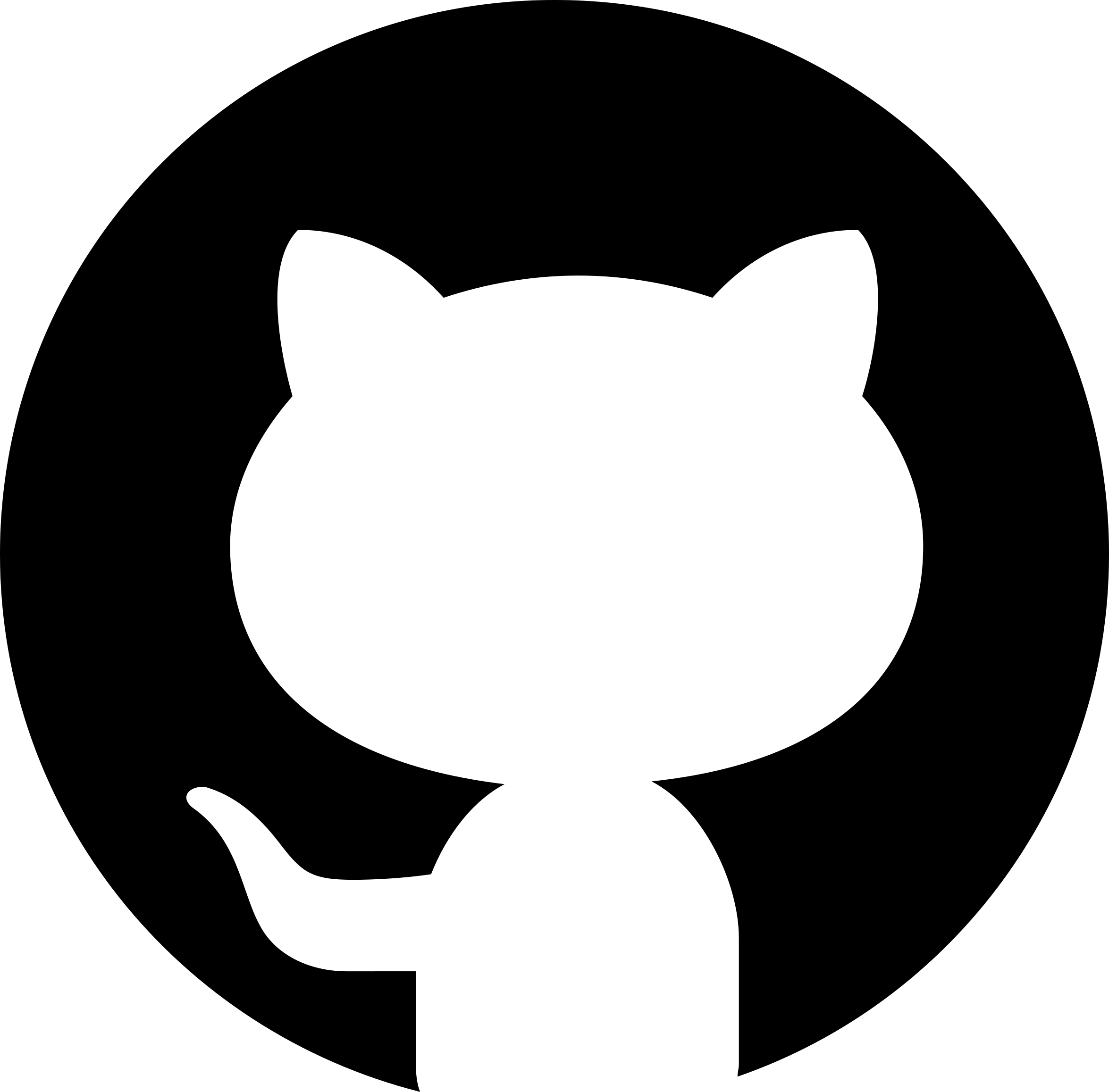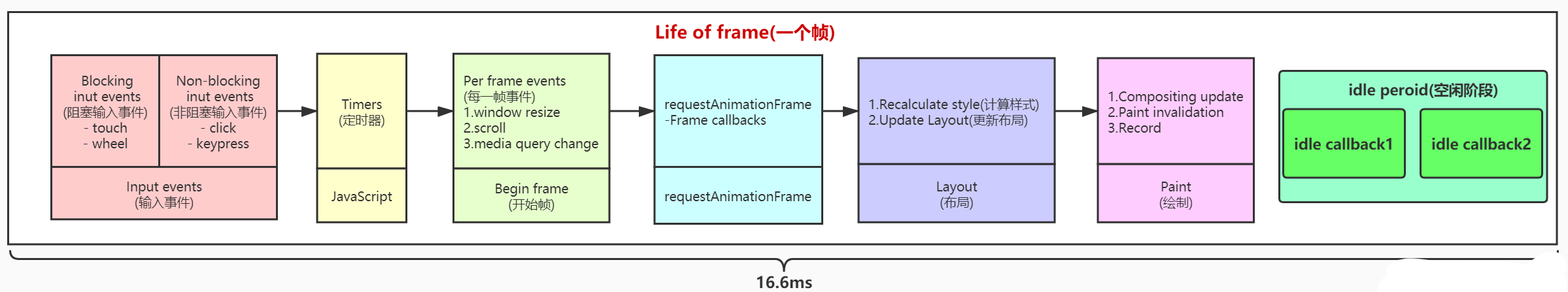# react fiberEdit on GitHub

#### what is react fiber

React Fiber is to increase its suitability for areas like animation, layout, and gestures. Its headline feature is incremental rendering: the ability to split rendering work into chunks and spread it out over multiple frames.

#### 关于 Fiber 你需要知道的基础知识

##### 浏览器刷新率（帧）

[]

1. 接受输入事件
2. 执行事件回调
3. 开始一帧
4. 执行 RAF (RequestAnimationFrame)
5. 页面布局，样式计算
6. 渲染
7. 执行 RIC (RequestIdelCallback)

##### JS 原生执行栈

React Fiber 出现之前，React 通过原生执行栈递归遍历 VDOM 浏览器引擎会从执行栈的顶端开始执行，执行完毕就弹出当前执行上下文，开始执行下一个函数，直到执行栈被清空才会停止。然后将执行权交还给浏览器。由于 React 将页面视图视作一个个函数执行的结果。每一个页面往往由多个视图组成，这就意味着多个函数的调用。

##### 时间切片（Time Slicing）

examble：曹冲称象

`let list = document.querySelector('.list')let total = 100000for (let i = 0; i < total; ++i) {    let item = document.createElement('li')    item.innerText = `我是\${i}`    list.appendChild(item)}`

```let list = document.querySelector('.list')let total = 100000let onceNumber = 20let currentIndex = 0
const renderToPage = (total, currentIndex) => {if(total <= 0){    return}let loopNumber = Math.min(total, onceNumber);
window.requestAnimationFrame(() => {    let fragment = document.createDocumentFragment();    for (let i = 1; i <= loopNumber; i++) {    let item = document.createElement('li')            item.innerText = `我是 new \${currentIndex + i}`    fragment.appendChild(item)    }    list.appendChild(fragment)
let newTotal = total - loopNumber    let newIndex = currentIndex + loopNumber    if(newTotal > 0){    renderToPage(newTotal, newIndex)  }})
}
renderToPage(total, currentIndex)```